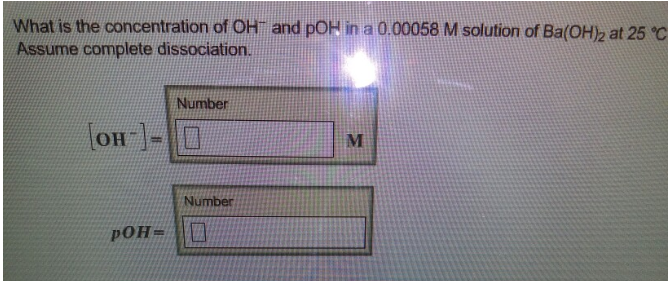Chemistry Practice Problems pH and pOH Practice Problems Solution: What is the concentration of OH- and pOH in a 0.00...

🤓 Based on our data, we think this question is relevant for Professor Smeureanu's class at HUNTER.

# Solution: What is the concentration of OH- and pOH in a 0.00058 M solution of Ba(OH)2 at 25°. Assume complete dissociation. [OH-] =pOH =

###### Problem

What is the concentration of OH- and pOH in a 0.00058 M solution of Ba(OH)2 at 25°. Assume complete dissociation.

[OH-] =
pOH =View Complete Written Solution

pH and pOH

pH and pOH

#### Q. What are the H3O+ and OH- concentrations of solutions that have the following pH values? Part ApH3 Express your answer using one significant figure se...

Solved • Tue Aug 21 2018 14:48:55 GMT-0400 (EDT)

pH and pOH

#### Q. A solution of NaOH(aq) contains 6.6 g of NaOH(s) per 100.0 mL of solution. Calculate the pH and the pOH of the solution at 25°C. pH = pOH =

Solved • Tue Aug 14 2018 13:03:26 GMT-0400 (EDT)

pH and pOH

#### Q. Calculate [H3O+] for the following solutions:a. 3.44 x 10-3 M HBr[H3O+] = b. 1.50 x 10-2 M KOH[H3O+] =

Solved • Tue Aug 14 2018 12:38:02 GMT-0400 (EDT)

pH and pOH

#### Q. Calculate the pH of an aqueous solution that is 0.020 M in HCl (aq) at 25°C.

Solved • Tue Aug 14 2018 11:53:21 GMT-0400 (EDT)